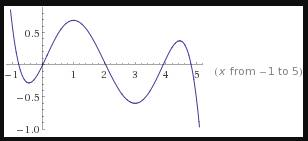# a = 2 To sketch: The graph of the function where values of function are given at some points.### Single Variable Calculus: Concepts...

4th Edition
James Stewart
Publisher: Cengage Learning
ISBN: 9781337687805### Single Variable Calculus: Concepts...

4th Edition
James Stewart
Publisher: Cengage Learning
ISBN: 9781337687805

#### Solutions

Chapter 2.6, Problem 22E
To determine

## a=2To sketch:The graph of the function where values of function are given at some points.

Expert Solution

Value of the function and derivative of the function at are 3 and 4 respectively.

### Explanation of Solution

Given: y=g(x) and g(0)=g(2)=g(4)=0,

g'(1)=g'(3)=0,g'(0)=g'(4)=1

g'(2)=1,limxg(x)=, and limxg(x)=

Let g(x)=ax2+bx+c be any function.

It has to find the graph and equation of the function.

g(x)=0 For x=0,2,4(given)

g'(x)=0 For x=1,3

g'(x)=1 For x=0,4 and g'(2)=1

Now

g'(x)=16(x1)(x3)(6(x2)2)

Integrate with respect to x then g(x)

g(x)=16(x1)(x3)(6(x2)2)dx=x530+x4317x318+x33+x

Graph of g(x)=x530+x4317x318+x33+x is given belowHence, the function is g(x)=x530+x4317x318+x33+x .

### Have a homework question?

Subscribe to bartleby learn! Ask subject matter experts 30 homework questions each month. Plus, you’ll have access to millions of step-by-step textbook answers!